Select Page

# EFL - ESL lesson plans for beginners | A1 Upper level

Welcome to our collection of ESL lesson plans and resources for teaching beginners. These lessons cover some of the fundamental grammar and functional areas of the English language and are aimed at younger learners, although they can be adapted and used with adults. Depending on the class size and level of your students, lessons should take between 60 and 90 minutes. Each lesson plan contains suggested board work, several low-prep classroom activities and a game.

If you find the free materials useful and want complete access to all of our teaching resources, you should consider becoming a member. Also, if you have an Android or iOS device, check out the TEFL Handbook app. We have designed it for use in the classroom, and it gives teachers offline access to all of our lesson plans.LESSON 1 | Functional language
Introductions
This ESL role-play lesson plan gives students a chance to practise giving introductions.
A1+LESSON 2 | Functional language
What's this?
In this lesson plan, students learn to ask and answer questions about their local environment using the correct articles.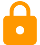A1+LESSON 3 | Grammar
Teaching plurals
ESL activities, games and resources for teaching plural nouns to beginners.
A1+LESSON 4 | Functional language
Giving instructions
This ESL lesson plan teaches beginners how to give basic instructions in English.
A1+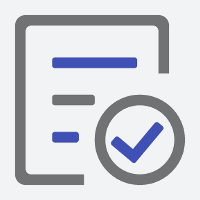LESSON 5 | Grammar
To be: Short forms
Students are introduced to the short forms of the verb 'to be' in this grammar centric lesson plan.A1+LESSON 6 | Grammar
To be: Questions
An ESL lesson plan that gives students a chance to practise asking questions using the verb 'to be'.A1+LESSON 7 | Grammar
This beginner-level ESL lesson plan serves as an excellent introduction to possessive adjectives.A1+LESSON 8 | Grammar
Possessive 's
In this grammar lesson plan, students learn how to use the possessive forms of different nouns.A1+LESSON 9 | Grammar
Have got
Students learn to work with the short forms of the verb 'have got' in this beginner-level ESL lesson plan.A1+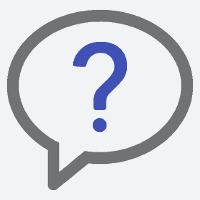LESSON 10 | Grammar
Have got: Questions
This ESL lesson plan builds on the previous lesson and introduces students to the question forms of the verb 'have got'.A1+LESSON 11 | Grammar
In this grammar lesson plan, students learn some of the basic rules around adjective position.A1+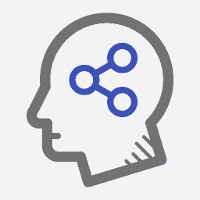LESSON 12 | Grammar
Can for ability
Students learn to express their abilities to their classmates through these fun ESL writing and speaking activities.A1+LESSON 13 | Functional language
Describing people
This lesson plan contains activities and games for teaching beginners how to describe people using the verbs 'to be' and 'have got'.A1+LESSON 14 | Functional language
Time
A collection of ESL activities and games that teach beginners how to tell the time in English.A1+LESSON 15 | Grammar
Prepositions of time
In this ESL lesson plan for beginners, students are taught how to use prepositions of time 'on' and 'at'.A1+LESSON 16 | Functional language
Fun and practical ESL activity and game ideas for teaching beginners how to ask questions about the time.A1+LESSON 17 | Grammar
Present simple
A beginner-level lesson plan that focuses on the grammar of the present simple tense.A1+LESSON 18 | Grammar
Present simple: Questions with do
This grammar-centric ESL lesson plan introduces some fundamental question structures of the present simple.A1+LESSON 19 | Grammar
Prepositions of place
In this fun ESL lesson plan, students learn to use prepositions of place to give richer descriptions of their environments.A1+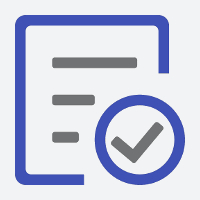LESSON 20 | Grammar
There is and there are
Students build on what they have learned to combine prepositions of place with the singular and plural forms of the verb to be.
A1+LESSON 21 | Grammar
Present continuous
This ESL lesson plan for beginners introduces students to the grammar of the present continuous tense.A1+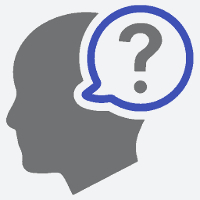LESSON 22 | Grammar
Present continuous: Questions
Students learn how to form questions in present continuous through these engaging ESL activities and games.A1+

We've put together a collection of ESL lesson plans and a mobile app that will reduce your planning time and improve the way you teach.